2. SOLUTION – LONG ANSWER TYPE QUESTIONS

Q. 1. Define the following terms :
(i) Mole fraction (ii) Molality (iii) Molarity (iv) Mass percentage.

Ans⇒ (i) Mole fraction : The ratio of the moles of a component A to be total moles of the solution is called the mole fraction of A. It is denoted by symbol x. For a binary solution of components A and B.
Mole fraction of A = XA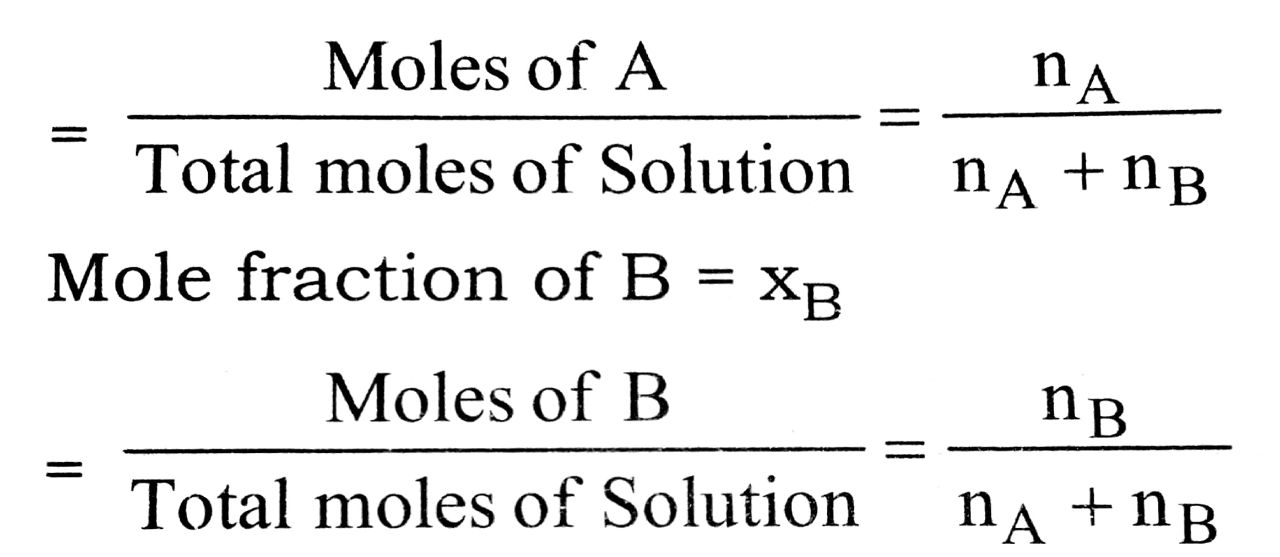(ii) Molality : The moles of the solute dissolved in one kilogram of the solvent is called the molality of the solution.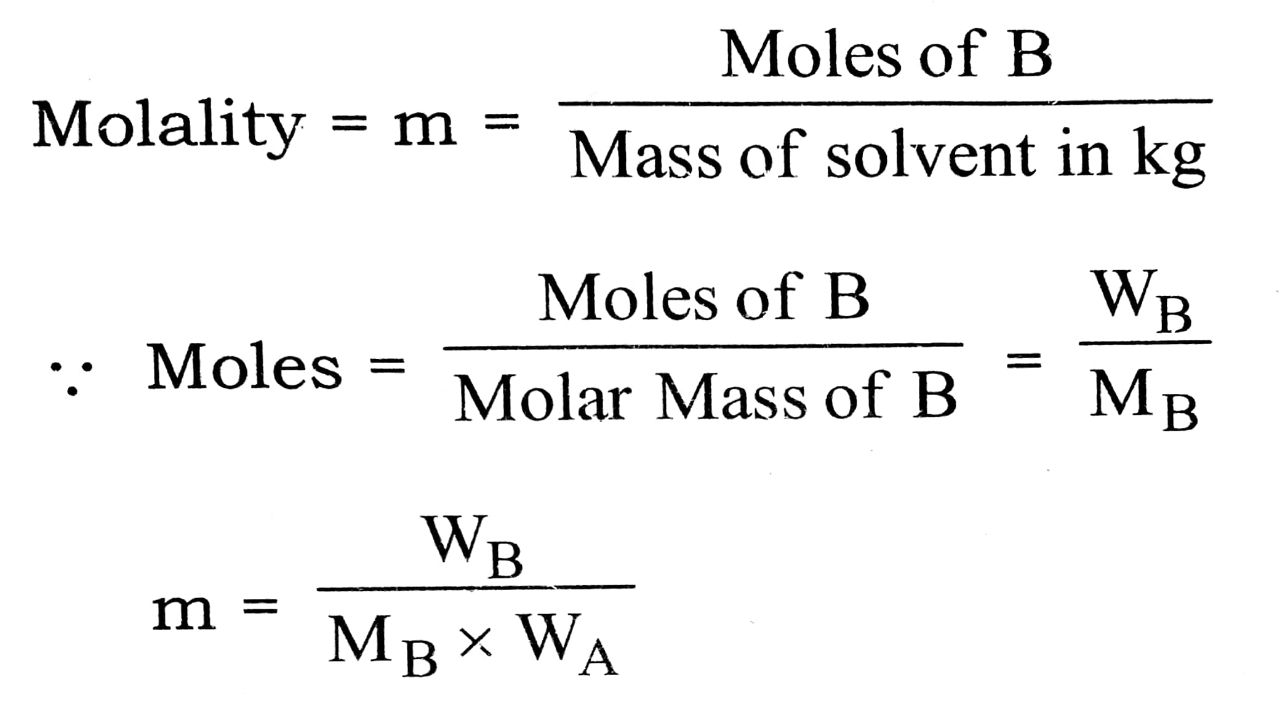where wA = Mass of solvent in kg.

(iii) Molarity : The concentration expressed as the moles of solute per litre of solution is the molarity. It is denoted by M.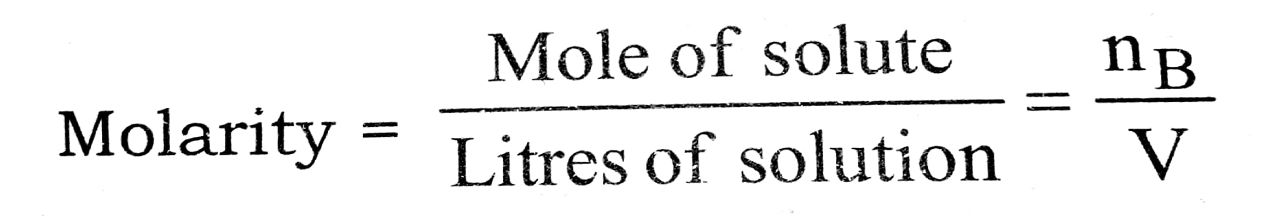A solution of one molar concentration is represented by 1 M, a two molar solution by 2 M and a one tenth molar solar solution is represented by 0.1 M.

(iv) Mass percentage : It is the amount of solute in gram’s dissolved per 100 g of solution. e.g. 5% solution sodium carbonate mass 5 g of solid sodium carbonate is present is 100 g of solution.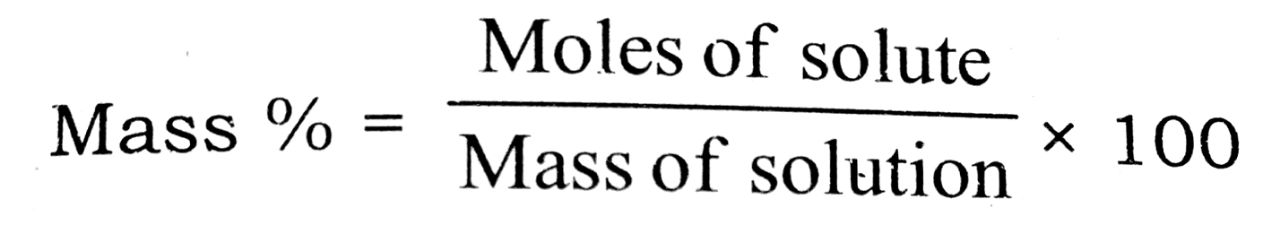Q. 2. Which type of deviation is shown by the solution formed by mixing cyclohexane and ethanol ?

Ans⇒ Hydrogen bonds exist among the molecules of ethanol (i.e., strong forces). When cyclohexane is added to ethanol, the cyclohexane molecule will come in between the ethanol molecules and disturb the hydrogen bondings. The forces between ethanol and cyclohexane will be weaker than the previous forces (H-bonding), hence there will be positive deviation.

Q. 3. (a) What is reverse osmosis ?

(b) What is the boiling point of glucose solution containing 18gm of glucose in 100 gm of H2O ? Molar elevation constant of H2O is 0.52 k kg mol-1.

Ans. (a) Reverse osmosis : If external pressure greater than osmotic pressure applied on solution, the flow of solvent molecules can be made proceed from the solution towards pure solvent. This type of osmosis is termed as reverse osmosis. Reverse osmosis is used for the distilation of sea water for getting fresh drinking water.

(b) We know that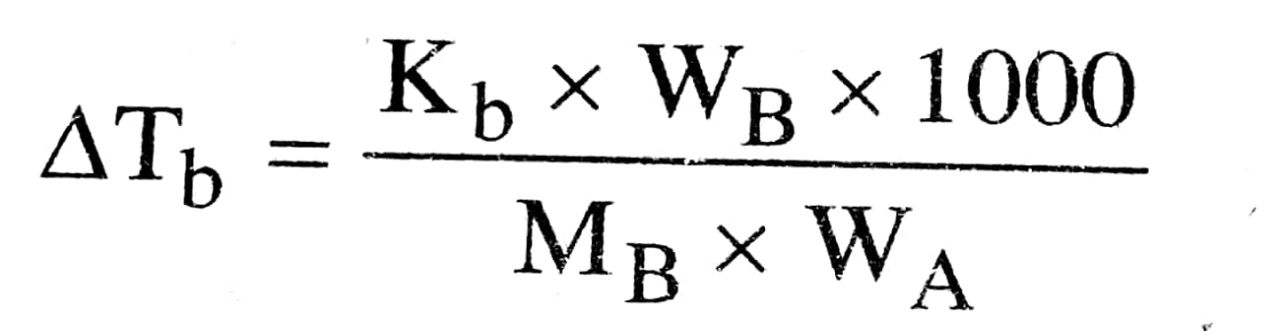MBWA Molecular wt. of glucose (C6H12O6) = 180 gram
Wt. of Glucose (WB) = 18 gram
Wt. of water (WA) = 100 gram

Q. 4. What do you mean by abnormal molecular mass ? Explain the factors with suitable examples which bring about the abnormality ?

Ans⇒ Molecular masses of the solutes which are inversely proportional to the colligative properties must have different values from their normal values. In other words, these are abnormal in nature and also called observed molecular masses.

In certain solvents (generally non-polar) the particles of the solute undergo association i.e., two or more molecules of solute combine to form a bigger molecule. As a result, the total number of

nA                                            ⇌                              (A).S
(Single molecules)                                     (Associated molecules)

Solute molecules in the solution decrease. The colligative properties are expected to decrease while the molecular masses of the solutes involved are expected to increase. It is unite expected also since the molecular mass associated above, varies inversely as the colligative property.

For example, in benzene solvent, both acetic acid and benzoic acid exist as dimers because of the presence of intemolecular hydrogen bonding.

2CH3COOH ⇌ (CH3COOH)2
2C6H5COOH ⇌ (C6H5COOH)2

KCl gives KI+ and Cl in aqueous solution hence the number of particles will be double in aqueous solution. There its abnormal molecular weight is half than normal molecular weight.

Q. 5. Define vapour pressure of a liquid. What happens to the vapour pressure when (a) volatile solute dissolves in the liquid and (b) the dissolved solute is non-volatile ?

Ans⇒ Every pure liquid exerts a vapour pressure in the space above it. This is the vapour pressure of the solvent over it at that particular temperature. It depende upon the nature of the solvent and the temperatura

(a) If a volatile solute is dissolved, vapour pressur of the solvent is increased.

(b) However, if a non-volatile solute is dissolved 11 it, the vapour pressure of the solution is lowered. In is because, in a solution, the percentage of the volatilo solvent molecules, which only contributes towards vapour pressure is diminished.

Since, the solute molecules are non-volatile and show no measurable tendency to escape from the solute as vapour, consequently, the vapour pressure of a solution is always lower than that of its solvent.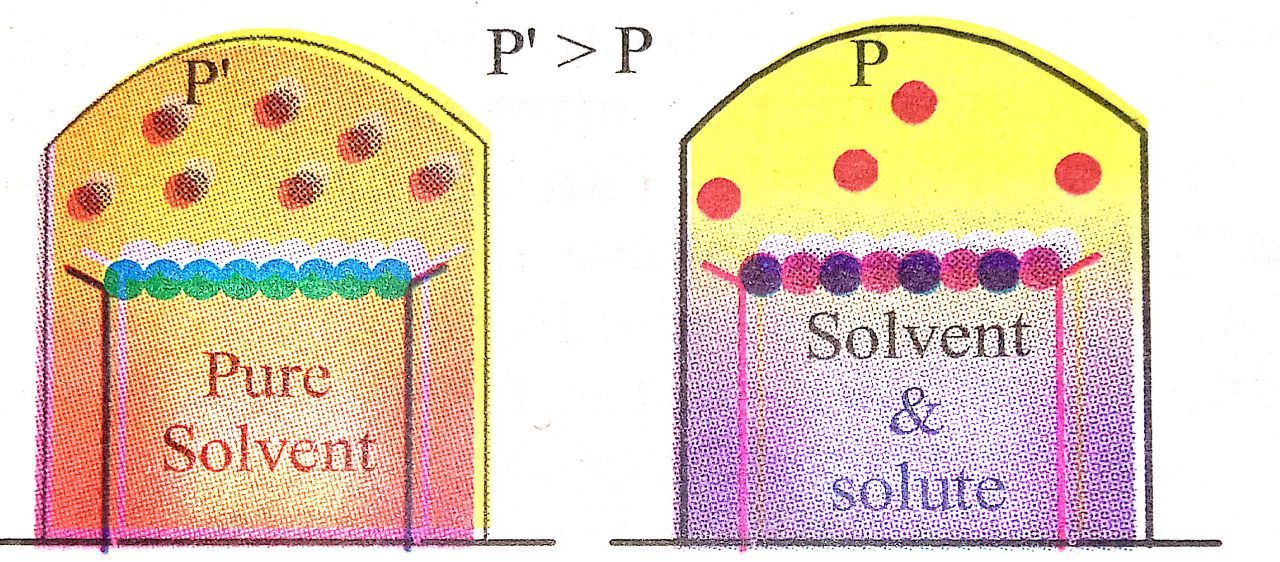Decrease in vapour pressure when a
non-volatile solute is added to the solvent.

Raoult’s law gave a relation between the relative lowering of vapour pressure and the mole fraction of the solute. Mathematically :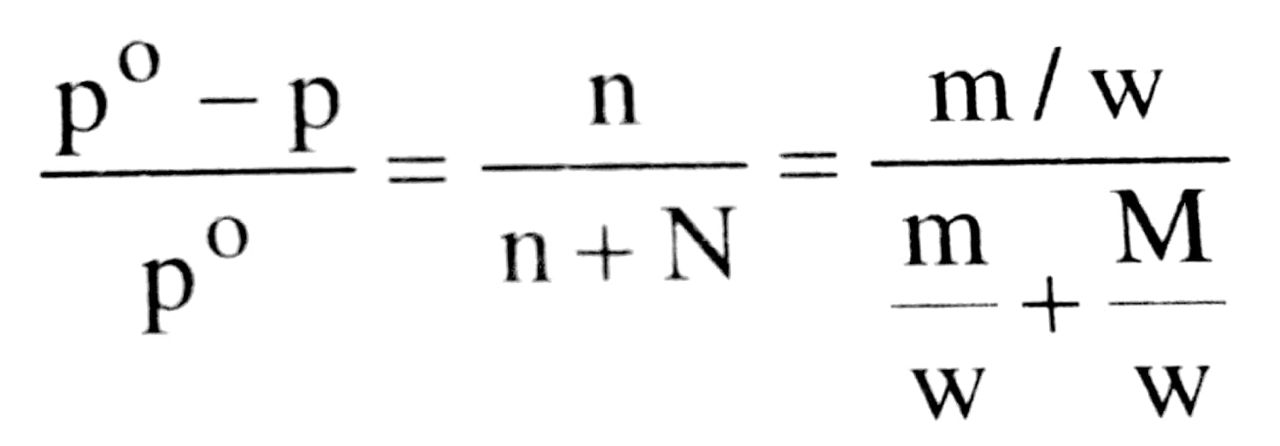(mole fraction of the solution)

Using the above equation, we can determine the molecular weight of the solute, when the lowering in v.p. is known, when a known weight of the solute w, dissolved in a known wt. of the solvent W. p॰ is the vapour pressure of the pure solvent and m and M are the molecular weights of solute and solvent respectively.

Q. 6. What is Rauolt’s law of relative lowering in vapour pressure (V.P.) ? Find out the molecular weight of non-volatile solute and also prove that molecular weight of solute is inversly proportional to lowering in V.P. ?

Ans⇒ Rauolts law of relative lowering of vapour pressure : When a non-volatile solute is added to pure solvent then vapour presure of solution is decreased. So according to Rauolt’s law relative lowering in vapour pressure is equal to mole fraction of solute.

Let P‍‌0 is the vapour pressure of pure solvent and WB is weight of non-volatile solute and Ps is the vapour pressure of solution then lowering in vapour pressure is P0 – PS or Δp.

Now according to Rauolt’s law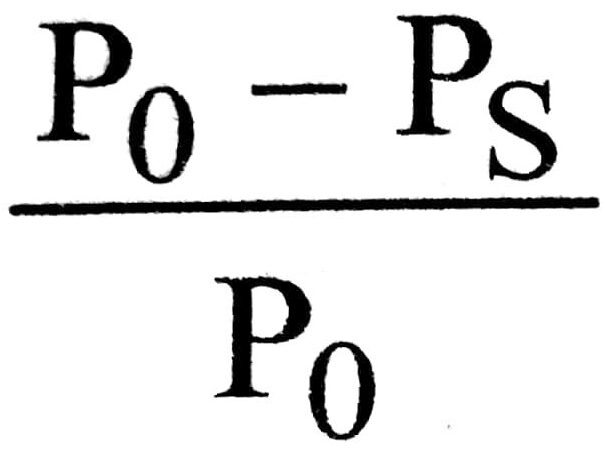= XB (Mole fraction of solute)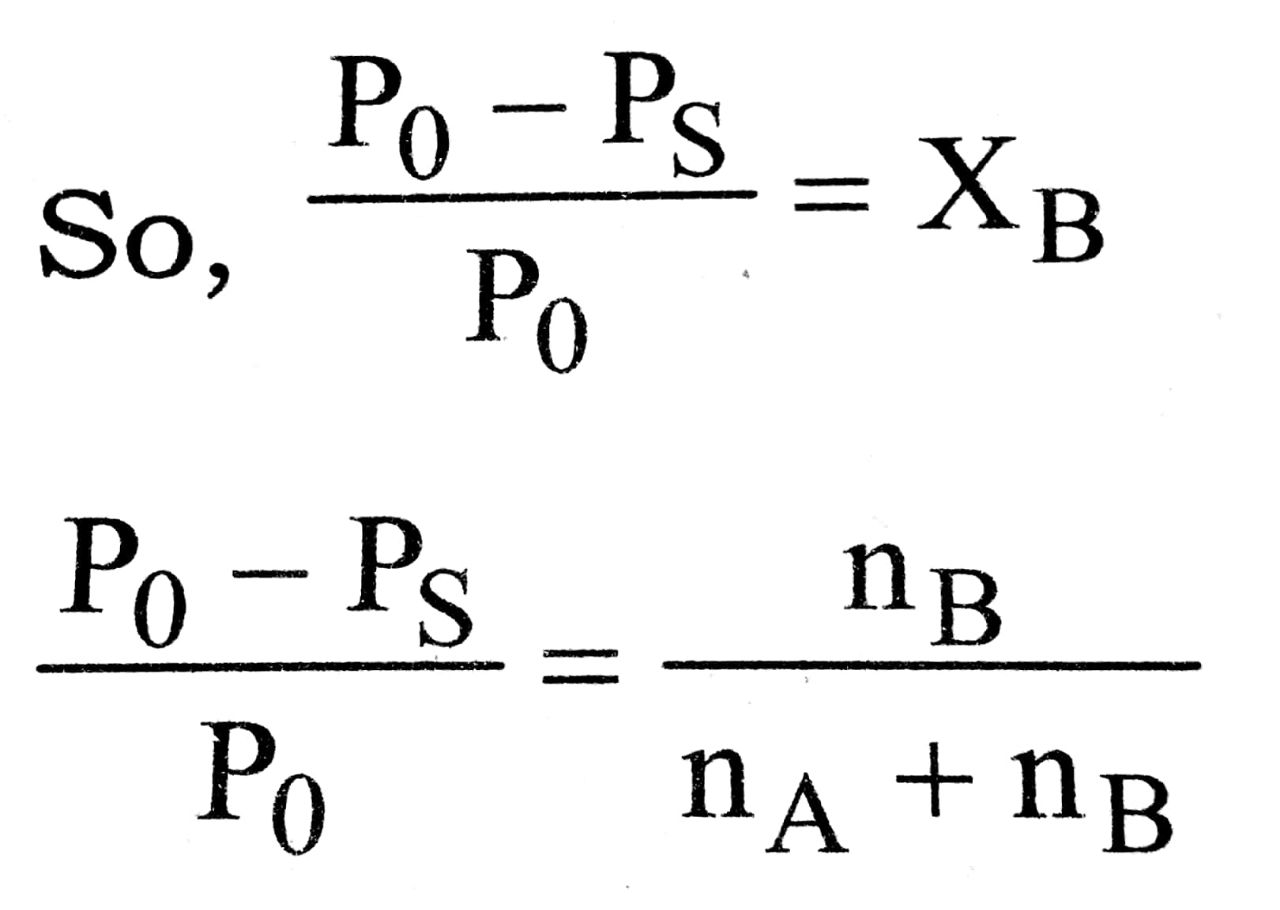where nA and nB are no. of moles of solvent and solute respectively.
For a very very dilute solution.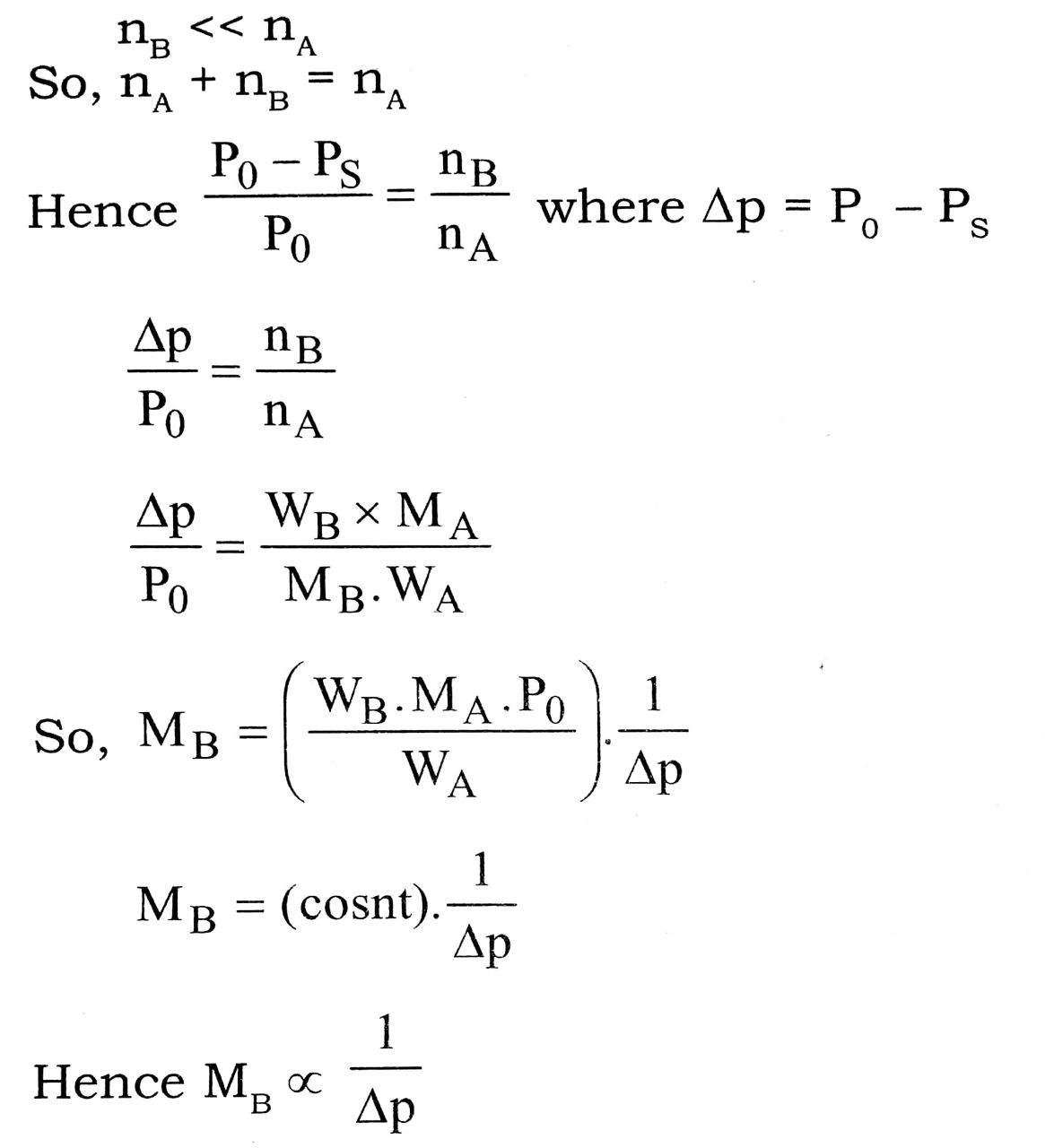Molecular weight of solute is inversely proportional to lowering in vapour pressure (V.P.)
where WB = weight of solute taken
MB = Molecular weight of solute
MA = molecular weight of solvent
Po – Ps = Δp = lowering in vapour pressure

Q. 7. State Henry’s law and mention some important applications.

Ans⇒ Henry’s Law : Effect of pressure on solubility of gases in liquids at a particular temperature is given by Henry’s law. “The mass of a gas dissolved in a given volume of the liquid at a constant temperature is directly proportional to the pressure of the gas present in equilibrium with the liquid.”

Mathematically m ∝ p
.                            m =  kp

where m = mass of the gas dissolved in a unit volume of the solvent

p = Pressure of the gas in equilibrium with the solvent

k = Constant called Henry’s Law constant.

Some important applications of Henry’s law :

1. To increase the solubility of CO2 in soft drinks and soda-water, the bottle is sealed under high pressure.

2. Deep sea divers use oxygen diluted with less soluble helium as breathing gas to minimise the painful effects accompanying the decompression.

3. In lungs where oxygen is present in air with high partial pressure, haemoglobin combines with oxygen to form oxohaemoglobin. In tissues where partial pressure of oxygen is low it releases oxygen for utilization in cellular activities.

Q. 16. The depression in freezing point of water observed for the same amount of acetic acid, trichloroacetic acid and trifluoroacetic acid increases in the order given above, Explain briefly.

Ans⇒ The lowering of vapour pressure of freezing point compared to that of the pure solvent. The freezing point of a Substance, the solid phase is in dynamic equilibrium with the liquid phase.

The freezing point of a substance, may be defined as the temperature at which the vapour pressure of the substance in its liquid phase is equal to its vapour pressure of solid phase. A solution will freeze when its vapour pressure equals the vapour pressure of the pure solid solvent.

According to Raoult’s law, when a non-volatic solid is added to the solvent its vapour decreases and it would become equal to that of solid solvent at lower temperature. The freezing point of the solvent decreases.

Let Tf॰ be the freezing point of pure solvent Tf be its freezing point when non-volatile solute is dissolved in it.

ΔTƒ = T॰ƒ – Tƒ depression of freezing point

Depression Constant : The unit of Kƒ is K kg. mol-1 values of Kf for some common solvent acetic acid are listed in this way. If W2 gram of the solute having molor mass as present in W1 gram of solvent produces the depression in freezing point ΔT1 of the solvent then molality,

The Solute is as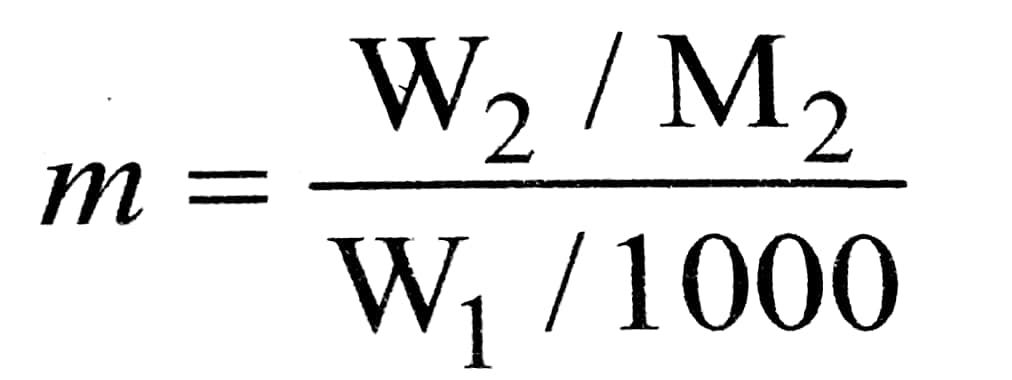We can find out that the freezing point for the same amount of acetic acid, trichloroacetic acid and trifluoroacetic acid increase in same order.

Q. 8. Define osmotic pressure. Prove that osmotic pressure is a molecular property.

Ans⇒ Osmotic pressure : The excess pressure is exerted on the surface of solution to just prevent osmosis is called osmotic pressure. It depends upon the molal concentration. Hence it is a colligative property.

According toVan’t Hoff π ∝ C (at constant temp.) and π ∝ T (At constant concentration).
Both laws are combined, we have

π ∝ CT and c =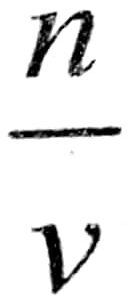or,       π    =   RCT
.           π     =RT
or,      πu   =   nRT
or,      π     =RT

where M = molecular weight and R = Gas constant.

## Class 12th Chemistry Long Type Question

 S.N CHEMISTRY LONG TYPE QUESTION 2022 1 SOLID STATE 2 SOLUTION 3 ELECTROCHEMISTRY 4 CHEMICAL KINETICS 5 SURFACE CHEMISTRY 6 GENERAL PRINCIPLES AND PROCESSES OF ISOLATION OF ELEMENTS 7 THE P-BLOCK ELEMENTS 8 THE D- AND f-BLOCK ELEMENTS 9 CO-ORDINATION COMPOUNDS 10 HALOALKANES AND HALOARENES 11 ALCOHOLS, PHENOLS AND ETHERS 12 ALDEHYDES, KETONES AND CARBOXYLIC ACIDS 13 AMINES 14 BIOMOLECULES 15 POLYMERS 16 CHEMISTRY IN EVERYDAY LIFE 17 DISTINGUISH BETWEEN PAIR 18 CONVERSIONS